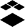cancel
Showing results for
Show  only  | Search instead for
Did you mean:

# New Quizzes: Grading for Multiple Answer Questions

I would like to see an additional grading option for Multiple Answer quiz questions in which the penalty points deducted for each incorrect answer = the total points for the question divided by the number of INCORRECT answers, rather than the number of correct answers.

This way overly harsh penalties won't be imposed in questions having more incorrect answer choices than correct ones, but the question score will still be zero if ALL answers are selected in an attempt to game the question.  Right now, the penalty point deduction calculation can harshly reduce such a question's score to zero even if all correct answers are chosen + just some incorrect answers.

This option should address concerns that many of my colleagues, other users, and students have raised about confusion and dissatisfaction with grading of such questions.  This option should be programmable using the same kind of algorithm as is presently used in the calculation of penalty points based on the number of correct answers.

Tags (5)Instructure Alumni
Status changed to: OpenCommunity Member

I would like to clarify our original request since there may be some confusion about it. The penalty deduction option we’re advocating does not eliminate giving (+) credit for correct answers. It merely adjusts the credit additionally deducted for incorrect answers in a more flexible way when the number of incorrect answer choices exceeds the number of correct answer choices.

For example, in a Multiple Answer question worth 12 points with 2 correct answers and 4 incorrect answers, here’s how the scenarios play out with the current score calculation algorithms—

(1) Only give credit for correct answers with no penalty for incorrect answers:

Each correct answer = 12 / 2 correct answers = 6 points. No deduction for incorrect answers.

Full credit can be obtained by selecting ALL answers = 6 + 6 = 12. This clearly games the system and should be avoided.

(2) Give credit for correct answers and deduct credit for incorrect answers:

Each correct answer = 12 / 2 correct answers = 6 points. Each incorrect answer = -12 / 2 correct answers = -6 points.

Selecting all answers gives 6 + 6 - 6 - 6 - 6 - 6 = -12, which would be rounded up to 0. Very harsh penalties.

Selecting 2 correct answers and only 2 incorrect answers gives 6 + 6 - 6 - 6 = 0. We would prefer this not to be zero, but rather something > 0, although not full credit. This combination entailed some thought by the quiz taker instead of just blindly choosing every answer with no thought. It seems that these two combinations should be scored differently. That is the problem with the current scoring algorithm—they're not scored differently.

So we propose this additional 3rd option—

(3) Give credit for correct answers and deduct credit for incorrect answers a different way:

Each correct answer = 12 / 2 correct answers = 6 points. Each incorrect answer = -12 / 4 INCORRECT answers = -3 points.

Selecting all answers gives 6 + 6 - 3 - 3 - 3 - 3 = 0, as desired. The system still can’t be gamed by blindly choosing every answer.

Selecting 2 correct answers and 2 incorrect answers gives 6 + 6 - 3 - 3 = 6. There is still a penalty for choosing some incorrect answers, but some credit is now given for correct answers. This combination is now distinguished from blindly choosing all answers.

This is the system we desire for questions with more incorrect answers than correct ones.

Please note that for questions with more correct answers than incorrect ones, option (2) would still allow the system to be partially gamed by choosing all the answers. Option (3) would give 0 score in that case and also prevent gaming. For anyone who feels that option (3)’s penalties are too harsh here, option (2) is still available.

The reason we advocate option (3) is that it can be programmed using basically the same algorithm calculations and interface as option (2). Just one calculation is altered and added, and one more checkbox is added.

Enabling the ability to assign individual credit to each answer choice would certainly be a wonderful addition, too, but it would require more new computation algorithms and user interface tools than option (3)’s and would entail much more programming. Option (3) is a quick addition that shouldn't require lots of programming overhead and would resolve lots of issues that have been raised concerning Multiple Answer scoring, especially with the Fall rolling around. Even if individual answer credit is eventually introduced, option (3) along with option (2) would still offer quick ways to engage their options without lots of individual points settings needed to be made manually.

Option (3) seems like a win-win addition.

I hope this helps clarify our request and reasoning. Thank-you for considering it.